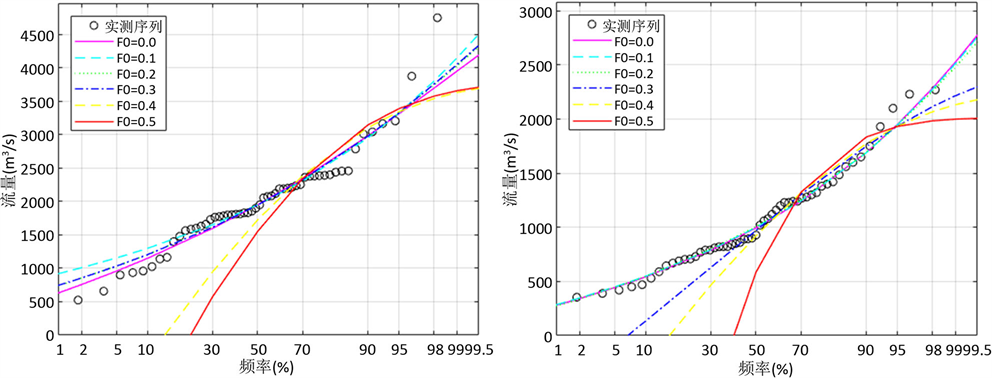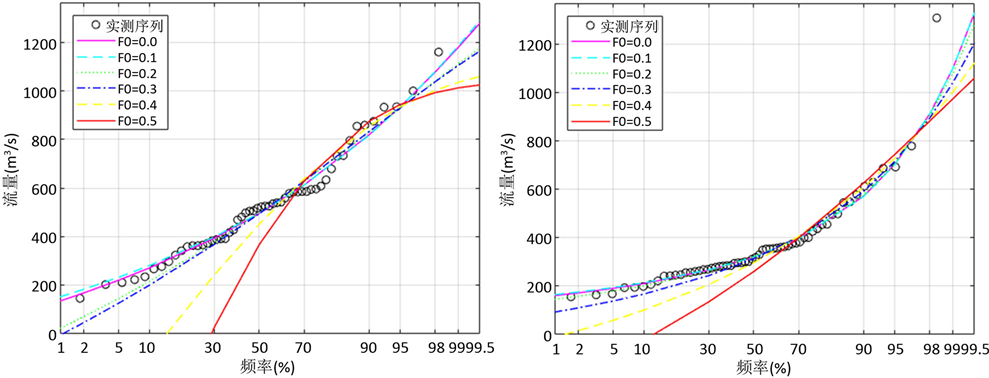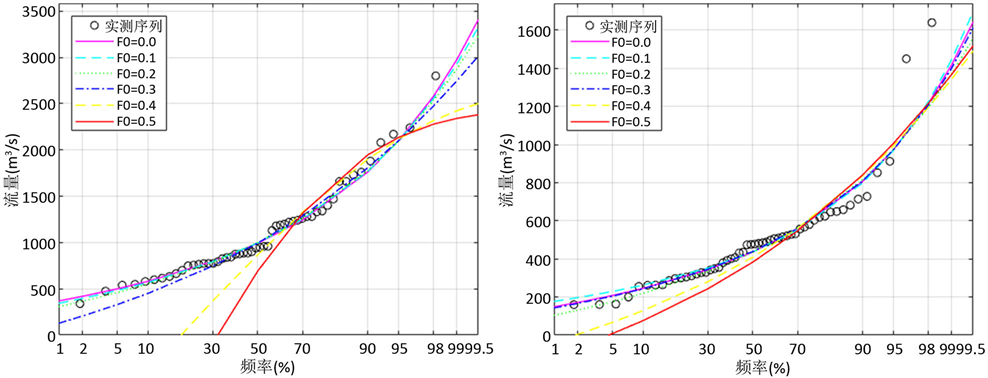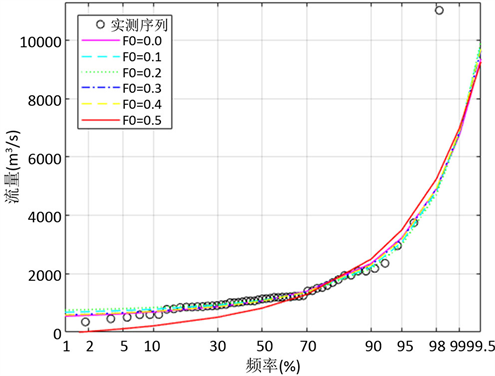﻿ 部分线性矩在黔南部地区洪水频率分析中的应用

部分线性矩在黔南部地区洪水频率分析中的应用The Application of Partial L-Moments for Flood Frequency Analysis in the South of Guizhou

Abstract: The south of Guizhou province suffers serious and frequent flood disasters. In order to provide an effi-cient and reliable theoretical basis for design of the flood control project in this area, the Partial L-moments are applied at the Bamao, Gaoche, Huishui, Guiyang, Libo, Caopingtou and Baben stations for flood frequency analysis. By estimating the parameters of Generalized Extreme Value (GEV) distribution and matching Partial L-Moments to annual maximum flow series of 7 hydrological stations, the design flood is calculated and the flood frequency curve is fitted. The cumulative squares error is used to evaluate the fitting ability of Partial L-moments and L-moments. The results show that as censored level F0 value increases, the relative deviation of the design value is smaller, except the hydrological stations of Gaoche and Caopingtou. Partial L-Moments can describe the data better in flood analysis and improve the estimation precision of design flood. Partial L-moments is a reasonable and effective method of flood frequency analysis in the south of Guizhou province.

1. 引言

2. 部分线性矩

${{\lambda }^{\prime }}_{1}=\frac{1}{{}^{n}C{}_{1}}\underset{i=1}{\overset{n}{\sum }}{x}_{\left(i\right)}^{*}$ (1)

${{\lambda }^{\prime }}_{2}=\frac{1}{2}\frac{1}{{}^{n}C{}_{2}}\underset{i=1}{\overset{n}{\sum }}\left({}^{i-1}C{}_{1}-{}^{n-i}C{}_{1}\right){x}_{\left(i\right)}^{*}$ (2)

${{\lambda }^{\prime }}_{3}\text{​}\text{​}\text{​}\text{​}\text{​}\text{​}=\frac{1}{3}\frac{1}{{}^{n}C{}_{3}}\underset{i=1}{\overset{n}{\sum }}\left({}^{i-1}C{}_{2}-2{}^{i-1}C{}_{1}{}^{n-i}C{}_{1}+{}^{n-i}C{}_{2}\right){x}_{\left(i\right)}^{*}$ (3)

${{\lambda }^{\prime }}_{4}=\frac{1}{4}\frac{1}{{}^{n}C{}_{4}}\underset{i=1}{\overset{n}{\sum }}\left({}^{i-1}C{}_{3}-3{}^{i-1}C{}_{2}{}^{n-i}C{}_{1}+3{}^{i-1}C{}_{1}{}^{n-i}C{}_{2}-{}^{n-i}C{}_{3}\right){x}_{\left(i\right)}^{*}$ (4)

${x}_{\left(i\right)}^{*}=\left\{\begin{array}{l}0\text{\hspace{0.17em}}\text{\hspace{0.17em}}\text{\hspace{0.17em}}\text{\hspace{0.17em}}\text{\hspace{0.17em}}\text{\hspace{0.17em}}\text{\hspace{0.17em}}{x}_{\left(i\right)}\le {x}_{0}\\ {x}_{\left(i\right)}\text{\hspace{0.17em}}\text{\hspace{0.17em}}\text{\hspace{0.17em}}\text{\hspace{0.17em}}\text{ }\text{ }{x}_{\left(i\right)}>{x}_{0}\end{array}$ (5)

${}^{n}C{}_{i}=\frac{n!}{i!\left(n-i\right)!}$ (6)

3. 广义极值分布及其部分线性矩

$F\left(x\right)=\left\{\begin{array}{l}\mathrm{exp}\left\{-{\left[1-\frac{k}{\alpha }\left(x-\xi \right)\right]}^{\frac{1}{k}}\right\};\text{\hspace{0.17em}}\text{\hspace{0.17em}}\text{ }\text{\hspace{0.17em}}\text{\hspace{0.17em}}\text{ }k\ne 0\\ \mathrm{exp}\left\{-\mathrm{exp}\left[-\frac{1}{\alpha }\left(x-\xi \right)\right]\right\};\text{\hspace{0.17em}}\text{\hspace{0.17em}}\text{\hspace{0.17em}}k=0\end{array}$ (7)

$x\left(F\right)=\left\{\begin{array}{l}\xi +\frac{\alpha }{k}\left[1-{\left(-\mathrm{ln}F\right)}^{k}\right];\text{\hspace{0.17em}}\text{\hspace{0.17em}}\text{\hspace{0.17em}}k\ne 0\\ \xi -\alpha \mathrm{ln}\left(-\mathrm{ln}F\right);\text{\hspace{0.17em}}\text{\hspace{0.17em}}\text{\hspace{0.17em}}\text{\hspace{0.17em}}\text{\hspace{0.17em}}\text{\hspace{0.17em}}\text{\hspace{0.17em}}\text{\hspace{0.17em}}\text{\hspace{0.17em}}\text{ }k=0\end{array}$ (8)

Wang (1990)  提出了GEV分布部分概率权重矩，当 $k\ne 0$ 时，其表达式为

${{\beta }^{\prime }}_{r}=\left(\xi +\frac{\alpha }{k}\right)\frac{1}{r+1}\left(1-{F}_{0}^{r+1}\right)-\frac{\alpha }{k}\frac{\Gamma \left(1+k\right)}{{\left(1+r\right)}^{1+k}}P\left(1+k,-\left(1+r\right)\mathrm{log}{F}_{0}\right)$ (9)

$P\left(1+k,-\left(1+r\right)\mathrm{log}{F}_{0}\right)={\int }_{0}^{-\left(1+r\right)\mathrm{log}{F}_{0}}\frac{{x}^{k}{\text{e}}^{-x}}{\Gamma \left(1+k\right)}\text{d}x$ (10)

$r=0,\text{1},\text{2}$ 代入式(9)，可得

${{\beta }^{\prime }}_{0}=\left(\xi +\frac{\alpha }{k}\right)\left(1-{F}_{0}\right)-\frac{\alpha }{k}\Gamma \left(1+k\right)P\left(1+k,-\mathrm{log}{F}_{0}\right)$ (11)

$\frac{2{{\beta }^{\prime }}_{1}}{1-{F}_{0}^{2}}-\frac{{{\beta }^{\prime }}_{0}}{1-{F}_{0}}=-\frac{\alpha }{k}\left[\frac{P\left(1+k,-2\mathrm{log}{F}_{0}\right)}{{2}^{k}\left(1-{F}_{0}^{2}\right)}-\frac{P\left(1+k,-\mathrm{log}{F}_{0}\right)}{1-{F}_{0}}\right]$ (12)

$\frac{\frac{2{{\beta }^{\prime }}_{1}}{1-{F}_{0}^{2}}-\frac{{{\beta }^{\prime }}_{0}}{1-{F}_{0}}}{\frac{3{{\beta }^{\prime }}_{2}}{1-{F}_{0}^{3}}-\frac{{{\beta }^{\prime }}_{0}}{1-{F}_{0}}}=\frac{\frac{P\left(1+k,-2\mathrm{log}{F}_{0}\right)}{{2}^{k}\left(1-{F}_{0}^{2}\right)}-\frac{P\left(1+k,-\mathrm{log}{F}_{0}\right)}{1-{F}_{0}}}{\frac{P\left(1+k,-3\mathrm{log}{F}_{0}\right)}{{3}^{k}\left(1-{F}_{0}^{3}\right)}-\frac{P\left(1+k,-\mathrm{log}{F}_{0}\right)}{1-{F}_{0}}}$ (13)

$z=\frac{\frac{2{{\beta }^{\prime }}_{1}}{1-{F}_{0}^{2}}-\frac{{{\beta }^{\prime }}_{0}}{1-{F}_{0}}}{\frac{3{{\beta }^{\prime }}_{2}}{1-{F}_{0}^{3}}-\frac{{{\beta }^{\prime }}_{0}}{1-{F}_{0}}}$ (14)

$z=\frac{\frac{P\left(1+k,-2\mathrm{log}{F}_{0}\right)}{{2}^{k}\left(1-{F}_{0}^{2}\right)}-\frac{P\left(1+k,-\mathrm{log}{F}_{0}\right)}{1-{F}_{0}}}{\frac{P\left(1+k,-3\mathrm{log}{F}_{0}\right)}{{3}^{k}\left(1-{F}_{0}^{3}\right)}-\frac{P\left(1+k,-\mathrm{log}{F}_{0}\right)}{1-{F}_{0}}}$ (15)

${F}_{0}\ne 0$ 时，给定k的取值范围 $-0.5\le k\le 0.5$ ，分别令 ${F}_{0}=0.1~0.5$ ，根据式(15)分别计算各组取值对应的z值，按式(16)拟合曲线，求得曲线拟合系数如表1所示。

$k={a}_{0}+{a}_{1}z+{a}_{2}{z}^{2}+{a}_{3}{z}^{3}+{a}_{4}{z}^{4}$ (16)

Hosking  (1990)给出线性矩与概率权重矩的前4阶关系为

${\lambda }_{1}={\beta }_{0}$ (17)

${\lambda }_{2}=2{\beta }_{1}-{\beta }_{0}$ (18)

${\lambda }_{3}=6{\beta }_{2}-6{\beta }_{1}+{\beta }_{0}$ (19)

${\lambda }_{4}=20{\beta }_{3}-30{\beta }_{2}+12{\beta }_{1}-{\beta }_{0}$ (20)

$z=\frac{\frac{{{\lambda }^{\prime }}_{2}+{{\lambda }^{\prime }}_{1}}{1-{F}_{0}^{2}}-\frac{{{\lambda }^{\prime }}_{1}}{1-{F}_{0}}}{\frac{\frac{1}{2}\left({{\lambda }^{\prime }}_{3}+3{{\lambda }^{\prime }}_{2}+2{{\lambda }^{\prime }}_{1}\right)}{1-{F}_{0}^{3}}-\frac{{{\lambda }^{\prime }}_{1}}{1-{F}_{0}}}$ (21)

$\stackrel{^}{z}=\frac{\frac{{{\stackrel{^}{\lambda }}^{\prime }}_{2}+{{\stackrel{^}{\lambda }}^{\prime }}_{1}}{1-{F}_{0}^{2}}-\frac{{{\stackrel{^}{\lambda }}^{\prime }}_{1}}{1-{F}_{0}}}{\frac{\frac{1}{2}\left({{\stackrel{^}{\lambda }}^{\prime }}_{3}+3{{\stackrel{^}{\lambda }}^{\prime }}_{2}+2{{\stackrel{^}{\lambda }}^{\prime }}_{1}\right)}{1-{F}_{0}^{3}}-\frac{{{\stackrel{^}{\lambda }}^{\prime }}_{1}}{1-{F}_{0}}}$ (22)$\stackrel{^}{\alpha }=-\frac{\stackrel{^}{k}}{\Gamma \left(1+\stackrel{^}{k}\right)}\frac{\frac{{{\stackrel{^}{\lambda }}^{\prime }}_{2}+{{\stackrel{^}{\lambda }}^{\prime }}_{1}}{1-{F}_{0}^{2}}-\frac{{{\stackrel{^}{\lambda }}^{\prime }}_{1}}{1-{F}_{0}}}{\frac{P\left(1+k,-2\mathrm{log}{F}_{0}\right)}{{2}^{k}\left(1-{F}_{0}^{2}\right)}-\frac{P\left(1+k,-\mathrm{log}{F}_{0}\right)}{1-{F}_{0}}}$ (23)

$\stackrel{^}{\beta }=\frac{{{\stackrel{^}{\lambda }}^{\prime }}_{1}}{1-{F}_{0}}+\frac{\stackrel{^}{\alpha }}{\stackrel{^}{k}}\left[\Gamma \left(1+\stackrel{^}{k}\right)\frac{P\left(1+k,-\mathrm{log}{F}_{0}\right)}{1-{F}_{0}}-1\right]$ (24)

${F}_{0}=0.0$ 时，部分线性矩转化为普通线性矩。Hosking  (1990)推出了GEV分布下普通线性矩为

${\lambda }_{1}=\xi +\alpha \left[1-\Gamma \left(1+k\right)\right]/k$ (25)

${\lambda }_{2}=\alpha \left(1-{2}^{-k}\right)\Gamma \left(1+k\right)/k$ (26)

${\tau }_{3}=2\left(1-{3}^{-k}\right)/\left(1-{2}^{-k}\right)-3$ (27)

${\tau }_{4}=\left[5\left(1-{4}^{-k}\right)-10\left(1-{3}^{-k}\right)+6\left(1-{2}^{-k}\right)\right]/\left(1-{2}^{-k}\right)$ (28)

$-0.5<{\tau }_{3}<0.5$ 时，三个参数的估计量计算公式分别为

$\stackrel{^}{k}=7.8590C+2.9554{C}^{2}$ (29)

$\stackrel{^}{\alpha }={\lambda }_{2}\stackrel{^}{k}/\left[\left(1-{2}^{-\stackrel{^}{k}}\right)\Gamma \left(1+\stackrel{^}{k}\right)\right]$ (30)

$\stackrel{^}{\xi }={\lambda }_{1}-\stackrel{^}{\alpha }\left[1-\Gamma \left(1+\stackrel{^}{k}\right)\right]/\stackrel{^}{k}$ (31)

4. 实例应用

4.1. 绘制频率曲线

4.2. 拟合效果分析

$\delta =\underset{i=i|P=50%}{\overset{i|P=98%}{\sum }}{\left(\frac{{x}_{i}-{\stackrel{^}{x}}_{i}}{{x}_{i}}\right)}^{2}$ (32)Table 2. Lengths of annual maximum flows and comparison of quantile errors using different F0(a) 八茂 (b) 高车(c) 惠水 (d) 贵阳(e) 荔波 (f) 草坪头(g) 把本

Figure 1. Flood frequency plot of annual maximum flows in northern Shaanxi

5. 结论

 裘峰. 贵州成灾洪水的主要特征[J]. 贵州水力发电, 1998, 12(3): 19-21. QIU Feng. The types of disastrous floods in Guizhou. Guizhou Water Power, 1998, 12(3): 19-21. (in Chinese)

 谢平, 李析男, 许斌, 等. 基于希尔伯特–黄变换的非一致性洪水频率计算方法: 以西江大湟江口站为例[J]. 自然灾害学报, 2013, 1(22): 85-94. XIE Ping, LI Xinan, XU Bin, et al. Hydrological frequency calculation method of inconsistent flood series based on Hil-ber-Huang: A case study of Dahuangjiangkou station of Xijiang River. Journal of Natural Disasters, 2013, 1(22): 85-94. (in Chinese)

 詹道江, 徐向阳, 陈元芳. 工程水文学[M]. 北京: 中国水利水电出版社, 2011: 1-282. ZHAN Daojiang, XU Xiangyang, CHEN Yuanfang. Engineering hydrology. Beijing: China Water Power Press, 2011: 1-282. (in Chinese)

 王俊珍, 宋松柏. 部分线性矩在洪水频率分析中的应用[J]. 水力发电学报, 2015, 12(1): 1-10. WANG Junzhen, SONG Songbai. Study on application of partial L-moments to flood frequency analysis. Journal of Hydroelectric Engineering, 2015, 12(1): 1-10. ( in Chinese)

 王俊珍, 宋松柏. 具有历史洪水资料的期望矩法参数估计研究[J]. 水力发电学报, 2014, 33(2): 8-18. WANG Junzhen, SONG Songbai. Expected Moments Algorithm method for parameters estimation with consideration of historical flood. Journal of Hydroelectric Engineering, 2014, 33(2): 8-18. ( in Chinese)

 邓育仁, 丁晶, 韦雪艳. 水文计算中的模糊优化适线法[J]. 水电站设计, 1995, 11(4): 43-47. DENG Yuren, DING Jing, WEI Xueyan. Fuzzy optimal curve fitting method in frequency analysis. Design of Hydroelectric Power Station, 1995, 11(4): 43-47. (in Chinese)

 谢平, 郑泽权. 水文频率计算有约束加权适线法[J]. 武汉水利电力大学学报, 2000, 33(1): 49-52. XIE Ping, ZHENG Zequan. A constrained and weighted fitting method for hydrologic frequency calculation. Journal of Wuhan University of Hydraulic and Electric Engineering, 2000, 33(1): 49-52. ( in Chinese)

 周芬, 郭生练, 肖义, 等. P-III型分布参数估计方法的比较研究[J]. 水电能源科学, 2003, 21(3): 10-13. ZHOU Fen, GUO Shenglian, XIAO Yi, et al. Comparative study on parameter estimation methods of Pearson type III distribution. Water Resource and Power, 2003, 21(3): 10-13. (in Chinese)

 BHATTARAI, K. P. Partial L-moments for the analysis of censored flood samples. Hydrological Sciences, 2004, 49(5): 855-868.
https://doi.org/10.1623/hysj.49.5.855.55138

 WANG, Q. J. Using partial probability weighted moments to fit the extreme value distributions to censored samples. Water Resources Research, 1996, 32(6): 1767-1771.
https://doi.org/10.1029/96WR00352

 WANG, Q. J. Estimation of GEV distribution from censored samples by method of partial probability weighted moments. Journal of Hydrology, 1990, 120: 103-114.
https://doi.org/10.1016/0022-1694(90)90144-M

 HOSKING, J. R. M. L-moments: Analysis and estimation of distributions using linear combinations of order statistics. Journal Royal Statistical Society, 1990, 52(2): 105-124.

Top